# NEET Questions Solved

The final product C, obtained in this reaction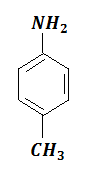$\stackrel{{\mathrm{Ac}}_{2}\mathrm{O}}{\to }$A$\underset{{\mathrm{CH}}_{2}\mathrm{COOH}}{\overset{{\mathrm{Br}}_{2}}{\to }}$B$\underset{{\mathrm{H}}^{+}}{\overset{{\mathrm{H}}_{2}\mathrm{O}}{\to }}$C

would be

(a)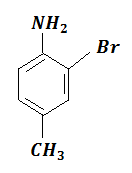(b)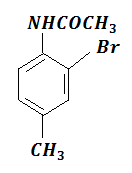(c)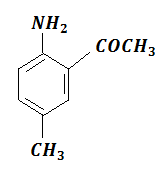(d)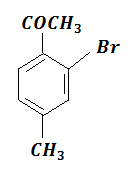Concept Videos :-

#1 | Preparation of Amines: I
#2 | Preparation of Amines: II
#3 | Properties of Amines: I
#4 | Properties of Amines: II

Concept Questions :-

Amines - Introduction,preparation and properties

(a)$\stackrel{{\mathrm{Ac}}_{2}\mathrm{O}}{\to }$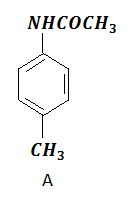$\underset{{\mathrm{CH}}_{2}\mathrm{COOH}}{\overset{{\mathrm{Br}}_{2}}{\to }}$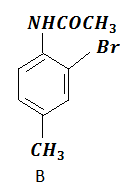$\underset{{\mathrm{H}}^{+}}{\overset{{\mathrm{H}}_{2}\mathrm{O}}{\to }}$Difficulty Level:

• 50%
• 30%
• 14%
• 8%
Crack NEET with Online Course - Free Trial (Offer Valid Till September 23, 2019)